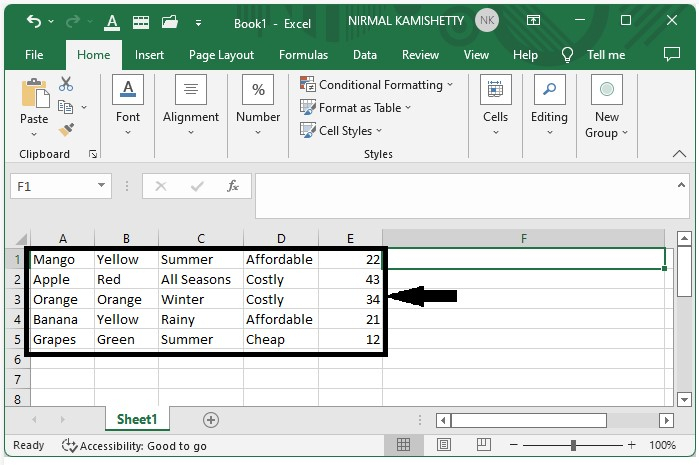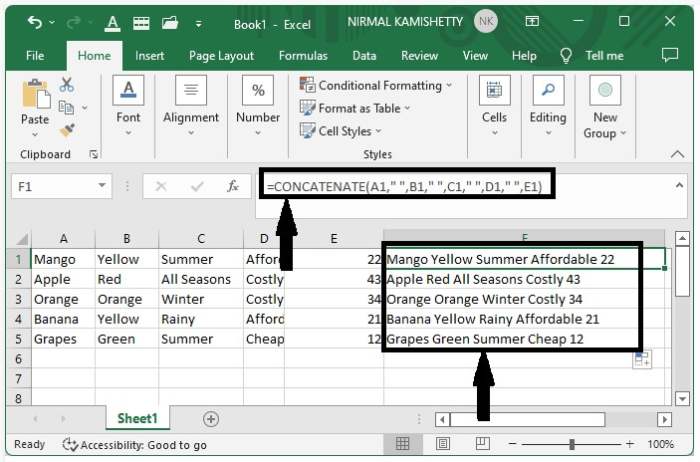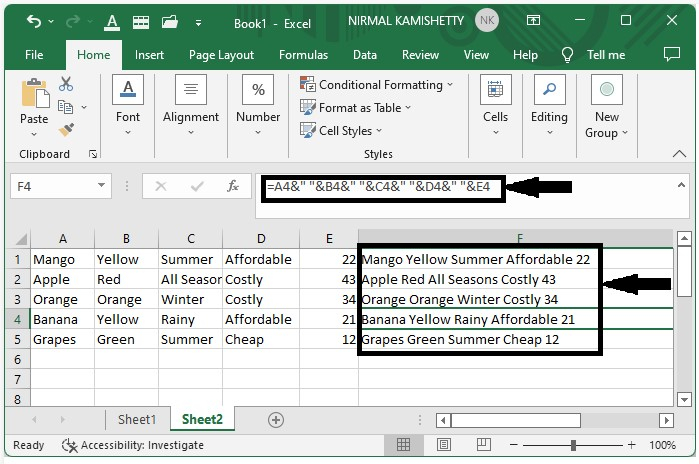# How to Append Text from One Cell to Another in Excel?

Consider the following scenario: we want to combine the text of multiple cells into a single cell. This is a very simple and commonly used method in Excel in order to perform activities in our day-to-day life usage of the program. This can be accomplished in two ways: by using the concatenation function or by inserting the "&" symbol between the cells. Read this tutorial to learn a simple process to add text from one cell to another in Excel.

## Appending Text from One Cell to Another using Concat

Here we will use the CONCAT formula to get any one of the results, then use the autofill handle to get all the results. Let us look at a simple process to see how we can add text from one cell to another in Excel.

Step 1

Let's consider an Excel sheet where the data is similar to the data shown in the below image.Step 2

Now, click on an empty box and enter the formula =CONCATENATE(A1, ", B1, ", C1, ", D1, ", E1) in the formula box and press enter to get the first result; we can also get all the other results by dragging down from the right corner until all the results are filled, and our result will look like the image below.## Appending Text from One Cell to Another using "&"

Here we will first get the first result using the & operation, then use the auto-fill handle to get all the results. Let us look at a simple process to see how we can add text from one cell to another in Excel.

Now, let us consider the same data which we used in the above. Now click on an empty box and enter the formula =A1&" "&B1&" "&C1&" "&D1&" "&E1 in the formula box and click on enter to get the first result. We can also get all the other results just by dragging down from the right corner till all the results are filled, and our result will look like the below screen shot shown below.## Conclusion

In this tutorial, we used a simple example to demonstrate how you can append or add text from one cell to another cell in Excel to highlight a particular set of data.